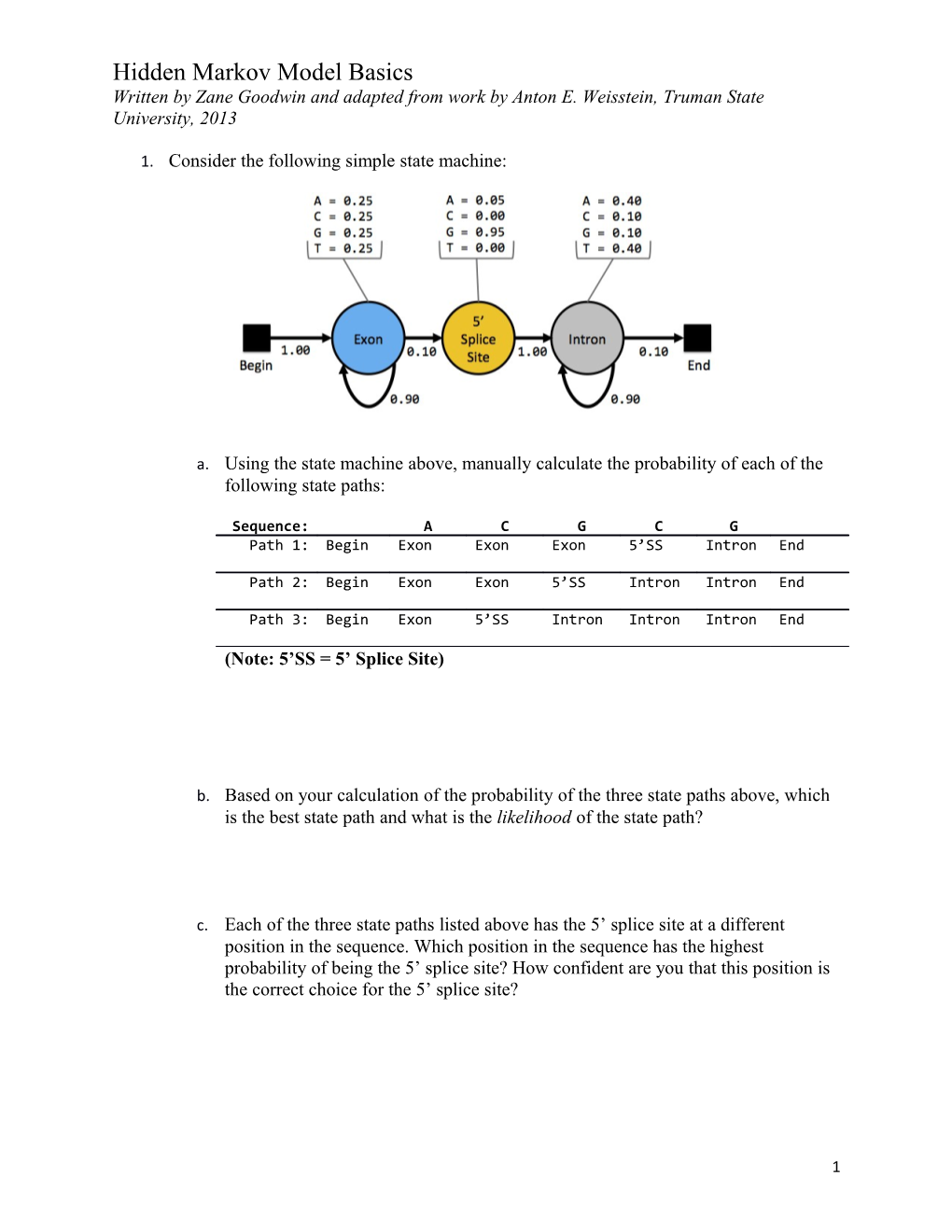# Note: 5 SS = 5 Splice Site1. Consider the following simple state machine:1. Using the state machine above, manually calculate the probability of each of the following state paths:

Sequence: / A / C / G / C / G
Path 1: / Begin / Exon / Exon / Exon / 5’SS / Intron / End
Path 2: / Begin / Exon / Exon / 5’SS / Intron / Intron / End
Path 3: / Begin / Exon / 5’SS / Intron / Intron / Intron / End

(Note: 5’SS = 5’ Splice Site)

1. Based on your calculation of the probability of the three state paths above, which is the best state path and what is the likelihood of the state path?
1. Each of the three state paths listed above has the 5’ splice site at a different position in the sequence. Which position in the sequence has the highest probability of being the 5’ splice site? How confident are you that this position is the correct choice for the 5’ splice site?
1. Given what you know about splice sites in eukaryotic genes, what are the assumptions made by this state machine that do not reflect the known characteristics of splice sites in eukaryotic genes?
1. Draw a state diagram for a Hidden Markov Model (HMM) that will scan a genomic sequence for a single gene that has one or more exons.
1. State Diagram:
1. What are the states in this Hidden Markov Model?
1. HMMs assume that each base is an independent observation. For instance, they assume that the probability of the current basebeing part of an intron does not affect whether the next base is part of the same intron. Give an example of a genomic feature where this assumption does not hold. Explain your reasoning.
1. For the next exercise, we will use an Excel workbook (HMM_intron.xls) to explore the properties of Hidden Markov Models. Before continuing with the rest of this exercise, please read the HMM Spreadsheet Manual to learn how to use this Excel workbook.

Make a copy of the Excel workbook and change the name of the workbook to HMM_intron_<your_initials>.xls (e.g. HMM_intron_ZG.xls). Then, answer the following questions using your copy of the workbook. This exercise will use the “Full Model” spreadsheet in the Excel workbook (click on the “Full Model” tab at the bottom toolbar to select this spreadsheet).

1. Use the first slider to change the exon transition probabilities to the following: Exon  Exon = 0.40, Exon  Splice = 0.60. Hold the exon transition probabilities constant and use the second slider to change the intron transition probabilities. How does this affect the likelihood profile of the 5’ splice site position (see the bar graph at the bottom of the spreadsheet)? Why does it have this effect?
1. Use the second slider to change the intron transition probabilities to the following: (Intron  Intron = 0.40, Intron  End = 0.60). Hold the intron transition probabilities constant and use the first slider to change the exon transition probabilities. How does this affect the likelihood profile of the 5’ splice site position? Why does it have this effect?
1. If your model predicts multiple positions in the sequence have the same likelihood to be a splice site, how could you use RNA-Seq data to identify the best splice site candidate?
1. QUESTION FOR THOUGHT: Many gene predictors use a collection of known genes (i.e. training set) to estimate the transition and emission probabilities in a HMM. For example, one can estimate the transition probabilities for the exon and intron states using the length distributions of exons and introns in the training set.
1. If the training set used to train a gene predictor contains many short genes and a few long genes, would you expect the HMM to predict more long genes or more short genes? Why?

(Note: Because the Excel workbook HMM_intron.xls models only part of a single gene, you cannot use the workbook to address this question.)

Last Update: 07/11/2013

1